1624907460

# Vim Tutorial. Step by Step

In this video, I try to teach important concepts I use in Vim. As the video progresses, it increases the difficulty of the concepts.

0:00 - Intro to Vim
3:15 - Modes in Vim
3:45 - Normal Mode
19:27 - Marking
22:03 - Insert Mode
25:18 - Splits
26:46 - Yanking and Pasting
27:37 - Search
29:06 - Visual Mode
29:48 - Copy to Clipboard
30:10 - Select block in Visual Mode
31:27 - to-upper-case and to-lower-case
31:51 - Replace Mode
33:41 - Move lines to the right
34:05 - Undo to the last save point
34:36 - Screen Movements
34:44 - Replace text
35:52 - Macros
41:42 - Vundle Plug-in Manager
42:57 - .vimrc and Vundle Install
44:35 - Installing a Plug-in using Vundle (NERDTree)
47:10 - Vim in VSCode

Don’t forget to Subscribe!

Share your ideas for my next video: https://request.manosriram.com
Find pair-programming partners: https://www.mydevfriend.com

Website: https://manosriram.com
Github: https://github.com/manosriram
My Blog: https://blog.manosriram.com

📻 Join the Discord Server: https://discord.gg/TaE9CTAmV9

#vim

## Buddha Community1561523460

## Matplotlib Cheat Sheet: Plotting in Python

This Matplotlib cheat sheet introduces you to the basics that you need to plot your data with Python and includes code samples.

Data visualization and storytelling with your data are essential skills that every data scientist needs to communicate insights gained from analyses effectively to any audience out there.

For most beginners, the first package that they use to get in touch with data visualization and storytelling is, naturally, Matplotlib: it is a Python 2D plotting library that enables users to make publication-quality figures. But, what might be even more convincing is the fact that other packages, such as Pandas, intend to build more plotting integration with Matplotlib as time goes on.

However, what might slow down beginners is the fact that this package is pretty extensive. There is so much that you can do with it and it might be hard to still keep a structure when you're learning how to work with Matplotlib.

DataCamp has created a Matplotlib cheat sheet for those who might already know how to use the package to their advantage to make beautiful plots in Python, but that still want to keep a one-page reference handy. Of course, for those who don't know how to work with Matplotlib, this might be the extra push be convinced and to finally get started with data visualization in Python.

You'll see that this cheat sheet presents you with the six basic steps that you can go through to make beautiful plots.

Check out the infographic by clicking on the button below: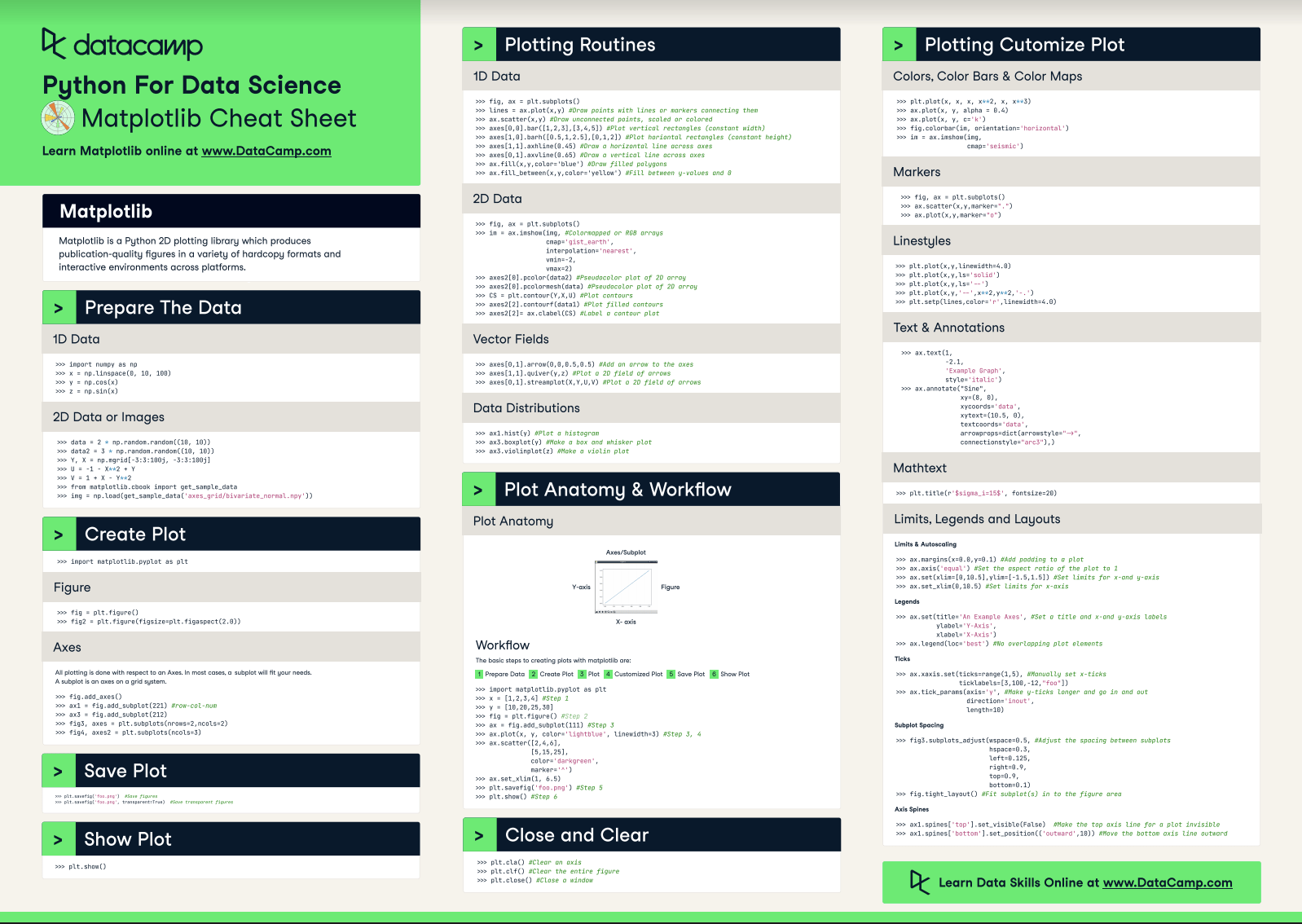With this handy reference, you'll familiarize yourself in no time with the basics of Matplotlib: you'll learn how you can prepare your data, create a new plot, use some basic plotting routines to your advantage, add customizations to your plots, and save, show and close the plots that you make.

What might have looked difficult before will definitely be more clear once you start using this cheat sheet! Use it in combination with the Matplotlib Gallery, the documentation.

Matplotlib

Matplotlib is a Python 2D plotting library which produces publication-quality figures in a variety of hardcopy formats and interactive environments across platforms.

## Prepare the Data

### 1D Data

``````>>> import numpy as np
>>> x = np.linspace(0, 10, 100)
>>> y = np.cos(x)
>>> z = np.sin(x)``````

### 2D Data or Images

``````>>> data = 2 * np.random.random((10, 10))
>>> data2 = 3 * np.random.random((10, 10))
>>> Y, X = np.mgrid[-3:3:100j, -3:3:100j]
>>> U = 1 X** 2 + Y
>>> V = 1 + X Y**2
>>> from matplotlib.cbook import get_sample_data

## Create Plot

``>>> import matplotlib.pyplot as plt``

### Figure

``````>>> fig = plt.figure()
>>> fig2 = plt.figure(figsize=plt.figaspect(2.0))``````

### Axes

``````>>> fig.add_axes()
>>> fig3, axes = plt.subplots(nrows=2,ncols=2)
>>> fig4, axes2 = plt.subplots(ncols=3)``````

## Save Plot

``````>>> plt.savefig('foo.png') #Save figures
>>> plt.savefig('foo.png',  transparent=True) #Save transparent figures``````

## Show Plot

``>>> plt.show()``

## 1D Data

``````>>> fig, ax = plt.subplots()
>>> lines = ax.plot(x,y) #Draw points with lines or markers connecting them
>>> ax.scatter(x,y) #Draw unconnected points, scaled or colored
>>> axes[0,0].bar([1,2,3],[3,4,5]) #Plot vertical rectangles (constant width)
>>> axes[1,0].barh([0.5,1,2.5],[0,1,2]) #Plot horiontal rectangles (constant height)
>>> axes[1,1].axhline(0.45) #Draw a horizontal line across axes
>>> axes[0,1].axvline(0.65) #Draw a vertical line across axes
>>> ax.fill(x,y,color='blue') #Draw filled polygons
>>> ax.fill_between(x,y,color='yellow') #Fill between y values and 0``````

### 2D Data

``````>>> fig, ax = plt.subplots()
>>> im = ax.imshow(img, #Colormapped or RGB arrays
cmap= 'gist_earth',
interpolation= 'nearest',
vmin=-2,
vmax=2)
>>> axes2.pcolor(data2) #Pseudocolor plot of 2D array
>>> axes2.pcolormesh(data) #Pseudocolor plot of 2D array
>>> CS = plt.contour(Y,X,U) #Plot contours
>>> axes2.contourf(data1) #Plot filled contours
>>> axes2= ax.clabel(CS) #Label a contour plot``````

### Vector Fields

``````>>> axes[0,1].arrow(0,0,0.5,0.5) #Add an arrow to the axes
>>> axes[1,1].quiver(y,z) #Plot a 2D field of arrows
>>> axes[0,1].streamplot(X,Y,U,V) #Plot a 2D field of arrows``````

### Data Distributions

``````>>> ax1.hist(y) #Plot a histogram
>>> ax3.boxplot(y) #Make a box and whisker plot
>>> ax3.violinplot(z)  #Make a violin plot``````

## Plot Anatomy & Workflow

### Plot Anatomy

y-axis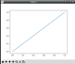x-axis

### Workflow

The basic steps to creating plots with matplotlib are:

1 Prepare Data
2 Create Plot
3 Plot
4 Customized Plot
5 Save Plot
6 Show Plot

``````>>> import matplotlib.pyplot as plt
>>> x = [1,2,3,4]  #Step 1
>>> y = [10,20,25,30]
>>> fig = plt.figure() #Step 2
>>> ax = fig.add_subplot(111) #Step 3
>>> ax.plot(x, y, color= 'lightblue', linewidth=3)  #Step 3, 4
>>> ax.scatter([2,4,6],
[5,15,25],
color= 'darkgreen',
marker= '^' )
>>> ax.set_xlim(1, 6.5)
>>> plt.savefig('foo.png' ) #Step 5
>>> plt.show() #Step 6``````

## Close and Clear

``````>>> plt.cla()  #Clear an axis
>>> plt.clf(). #Clear the entire figure
>>> plt.close(). #Close a window``````

## Plotting Customize Plot

### Colors, Color Bars & Color Maps

``````>>> plt.plot(x, x, x, x**2, x, x** 3)
>>> ax.plot(x, y, alpha = 0.4)
>>> ax.plot(x, y, c= 'k')
>>> fig.colorbar(im, orientation= 'horizontal')
>>> im = ax.imshow(img,
cmap= 'seismic' )``````

### Markers

``````>>> fig, ax = plt.subplots()
>>> ax.scatter(x,y,marker= ".")
>>> ax.plot(x,y,marker= "o")``````

### Linestyles

``````>>> plt.plot(x,y,linewidth=4.0)
>>> plt.plot(x,y,ls= 'solid')
>>> plt.plot(x,y,ls= '--')
>>> plt.plot(x,y,'--' ,x**2,y**2,'-.' )
>>> plt.setp(lines,color= 'r',linewidth=4.0)``````

### Text & Annotations

``````>>> ax.text(1,
-2.1,
'Example Graph',
style= 'italic' )
>>> ax.annotate("Sine",
xy=(8, 0),
xycoords= 'data',
xytext=(10.5, 0),
textcoords= 'data',
arrowprops=dict(arrowstyle= "->",
connectionstyle="arc3"),)``````

### Mathtext

``>>> plt.title(r '\$sigma_i=15\$', fontsize=20)``

### Limits, Legends and Layouts

Limits & Autoscaling

``````>>> ax.margins(x=0.0,y=0.1) #Add padding to a plot
>>> ax.axis('equal')  #Set the aspect ratio of the plot to 1
>>> ax.set(xlim=[0,10.5],ylim=[-1.5,1.5])  #Set limits for x-and y-axis
>>> ax.set_xlim(0,10.5) #Set limits for x-axis``````

Legends

``````>>> ax.set(title= 'An Example Axes',  #Set a title and x-and y-axis labels
ylabel= 'Y-Axis',
xlabel= 'X-Axis')
>>> ax.legend(loc= 'best')  #No overlapping plot elements``````

Ticks

``````>>> ax.xaxis.set(ticks=range(1,5),  #Manually set x-ticks
ticklabels=[3,100, 12,"foo" ])
>>> ax.tick_params(axis= 'y', #Make y-ticks longer and go in and out
direction= 'inout',
length=10)``````

Subplot Spacing

``````>>> fig3.subplots_adjust(wspace=0.5,   #Adjust the spacing between subplots
hspace=0.3,
left=0.125,
right=0.9,
top=0.9,
bottom=0.1)
>>> fig.tight_layout() #Fit subplot(s) in to the figure area``````

Axis Spines

``````>>> ax1.spines[ 'top'].set_visible(False) #Make the top axis line for a plot invisible
>>> ax1.spines['bottom' ].set_position(( 'outward',10))  #Move the bottom axis line outward``````

Have this Cheat Sheet at your fingertips

Original article source at https://www.datacamp.com

#matplotlib #cheatsheet #python1653464648

## Python Data Visualization: Bokeh Cheat Sheet

A handy cheat sheet for interactive plotting and statistical charts with Bokeh.

Bokeh distinguishes itself from other Python visualization libraries such as Matplotlib or Seaborn in the fact that it is an interactive visualization library that is ideal for anyone who would like to quickly and easily create interactive plots, dashboards, and data applications.

Bokeh is also known for enabling high-performance visual presentation of large data sets in modern web browsers.

For data scientists, Bokeh is the ideal tool to build statistical charts quickly and easily; But there are also other advantages, such as the various output options and the fact that you can embed your visualizations in applications. And let's not forget that the wide variety of visualization customization options makes this Python library an indispensable tool for your data science toolbox.

Now, DataCamp has created a Bokeh cheat sheet for those who have already taken the course and that still want a handy one-page reference or for those who need an extra push to get started.

In short, you'll see that this cheat sheet not only presents you with the five steps that you can go through to make beautiful plots but will also introduce you to the basics of statistical charts.

In no time, this Bokeh cheat sheet will make you familiar with how you can prepare your data, create a new plot, add renderers for your data with custom visualizations, output your plot and save or show it. And the creation of basic statistical charts will hold no secrets for you any longer.

Boost your Python data visualizations now with the help of Bokeh! :)

## Plotting With Bokeh

The Python interactive visualization library Bokeh enables high-performance visual presentation of large datasets in modern web browsers.

Bokeh's mid-level general-purpose bokeh. plotting interface is centered around two main components: data and glyphs.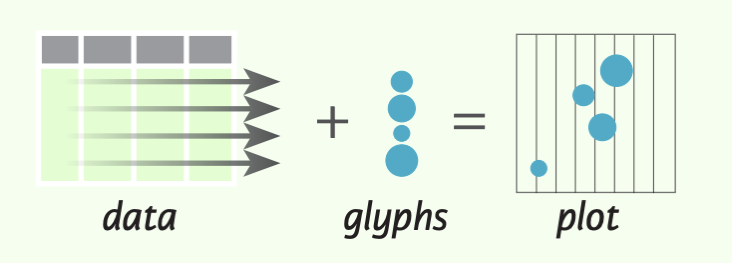The basic steps to creating plots with the bokeh. plotting interface are:

1. Prepare some data (Python lists, NumPy arrays, Pandas DataFrames and other sequences of values)
2. Create a new plot
4. Specify where to generate the output
5. Show or save the results
``````>>> from bokeh.plotting import figure
>>> from bokeh.io import output_file, show
>>> x = [1, 2, 3, 4, 5] #Step 1
>>> y = [6, 7, 2, 4, 5]
>>> p = figure(title="simple line example", #Step 2
x_axis_label='x',
y_axis_label='y')
>>> p.line(x, y, legend="Temp.", line_width=2) #Step 3
>>> output_file("lines.html") #Step 4
>>> show(p) #Step 5``````

## 1. Data

Under the hood, your data is converted to Column Data Sources. You can also do this manually:

``````>>> import numpy as np
>>> import pandas as pd
>>> df = pd.OataFrame(np.array([[33.9,4,65, 'US'], [32.4, 4, 66, 'Asia'], [21.4, 4, 109, 'Europe']]),
columns= ['mpg', 'cyl',   'hp',   'origin'],
index=['Toyota', 'Fiat', 'Volvo'])

>>> from bokeh.models import ColumnOataSource
>>> cds_df = ColumnOataSource(df)``````

## 2. Plotting

``````>>> from bokeh.plotting import figure
>>>p1= figure(plot_width=300, tools='pan,box_zoom')
>>> p2 = figure(plot_width=300, plot_height=300,
x_range=(0, 8), y_range=(0, 8))
>>> p3 = figure()``````

## 3. Renderers & Visual Customizations

### Glyphs

Scatter Markers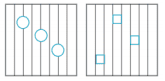``````>>> p1.circle(np.array([1,2,3]), np.array([3,2,1]), fill_color='white')
>>> p2.square(np.array([1.5,3.5,5.5]), [1,4,3],
color='blue', size=1)
``````

Line Glyphs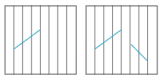``````>>> pl.line([1,2,3,4], [3,4,5,6], line_width=2)
>>> p2.multi_line(pd.DataFrame([[1,2,3],[5,6,7]]),
pd.DataFrame([[3,4,5],[3,2,1]]),
color="blue")
``````

### Customized Glyphs

Selection and Non-Selection Glyphs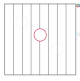``````>>> p = figure(tools='box_select')
>>> p. circle ('mpg', 'cyl', source=cds_df,
selection_color='red',
nonselection_alpha=0.1)
``````

Hover Glyphs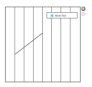``````>>> from bokeh.models import HoverTool
>>>hover= HoverTool(tooltips=None, mode='vline')
``````

Color Mapping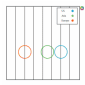``````>>> from bokeh.models import CategoricalColorMapper
>>> color_mapper = CategoricalColorMapper(
factors= ['US', 'Asia', 'Europe'],
palette= ['blue', 'red', 'green'])
>>>  p3. circle ('mpg', 'cyl', source=cds_df,
color=dict(field='origin',
transform=color_mapper), legend='Origin')
``````

## 4. Output & Export

### Notebook

``````>>> from bokeh.io import output_notebook, show
>>> output_notebook()
``````

### HTML

Standalone HTML

``````>>> from bokeh.embed import file_html
>>> from bokeh.resources import CON
>>> html = file_html(p, CON, "my_plot")

>>> from  bokeh.io  import  output_file,  show
>>> output_file('my_bar_chart.html',  mode='cdn')``````

Components

``````>>> from bokeh.embed import components
>>> script, div= components(p)``````

### PNG

``````>>> from bokeh.io import export_png
>>> export_png(p, filename="plot.png")``````

### SVG

``````>>> from bokeh.io import export_svgs
>>> p. output_backend = "svg"
>>> export_svgs(p,filename="plot.svg")``````

### Legend Location

Inside Plot Area

``````>>> p.legend.location = 'bottom left'
``````

Outside Plot Area

``````>>> from bokeh.models import Legend
>>> r1 = p2.asterisk(np.array([1,2,3]), np.array([3,2,1])
>>> r2 = p2.line([1,2,3,4], [3,4,5,6])
>>> legend = Legend(items=[("One" ,[p1, r1]),("Two",[r2])], location=(0, -30))

### Legend Background & Border

``````>>> p.legend. border_line_color = "navy"
>>> p.legend.background_fill_color = "white"``````

### Legend Orientation

``````>>> p.legend.orientation = "horizontal"
>>> p.legend.orientation = "vertical"``````

### Rows & Columns Layout

Rows

``````>>> from bokeh.layouts import row
>>>layout= row(p1,p2,p3)
``````

Columns

``````>>> from bokeh.layouts import columns
>>>layout= column(p1,p2,p3)``````

Nesting Rows & Columns

``````>>>layout= row(column(p1,p2), p3)
``````

### Grid Layout

``````>>> from bokeh.layouts import gridplot
>>> rowl = [p1,p2]
>>> row2 = [p3]
>>> layout = gridplot([[p1, p2],[p3]])``````

### Tabbed Layout

``````>>> from bokeh.models.widgets import Panel, Tabs
>>> tab1 = Panel(child=p1, title="tab1")
>>> tab2 = Panel(child=p2, title="tab2")
>>> layout = Tabs(tabs=[tab1, tab2])``````

``````Linked Axes
>>> p2.x_range = p1.x_range
>>> p2.y_range = p1.y_range``````

``````>>> p4 = figure(plot_width = 100, tools='box_select,lasso_select')
>>> p4.circle('mpg', 'cyl' , source=cds_df)
>>> p5 = figure(plot_width = 200, tools='box_select,lasso_select')
>>> p5.circle('mpg', 'hp', source=cds df)
>>>layout= row(p4,p5)``````

## 5. Show or Save Your Plots

``````>>> show(p1)
>>> show(layout)
>>> save(p1)``````

Have this Cheat Sheet at your fingertips

Original article source at https://www.datacamp.com

#python #datavisualization #bokeh #cheatsheet1596728880

## Tutorial: Getting Started with R and RStudio

In this tutorial we’ll learn how to begin programming with R using RStudio. We’ll install R, and RStudio RStudio, an extremely popular development environment for R. We’ll learn the key RStudio features in order to start programming in R on our own.

If you already know how to use RStudio and want to learn some tips, tricks, and shortcuts, check out this Dataquest blog post.

#data science tutorials #beginner #r tutorial #r tutorials #rstats #tutorial #tutorials1596513720

## 1. Characteristics of Clean Data and Messy Data

What exactly is clean data? Clean data is accurate, complete, and in a format that is ready to analyze. Characteristics of clean data include data that are:

• Free of duplicate rows/values
• Error-free (e.g. free of misspellings)
• Relevant (e.g. free of special characters)
• The appropriate data type for analysis
• Free of outliers (or only contain outliers have been identified/understood), and
• Follows a “tidy data” structure

Common symptoms of messy data include data that contain:

• Special characters (e.g. commas in numeric values)
• Numeric values stored as text/character data types
• Duplicate rows
• Misspellings
• Inaccuracies
• White space
• Missing data
• Zeros instead of null values

## 2. Motivation

In this blog post, we will work with five property-sales datasets that are publicly available on the New York City Department of Finance Rolling Sales Data website. We encourage you to download the datasets and follow along! Each file contains one year of real estate sales data for one of New York City’s five boroughs. We will work with the following Microsoft Excel files:

• rollingsales_bronx.xls
• rollingsales_brooklyn.xls
• rollingsales_manhattan.xls
• rollingsales_queens.xls
• rollingsales_statenisland.xls

As we work through this blog post, imagine that you are helping a friend launch their home-inspection business in New York City. You offer to help them by analyzing the data to better understand the real-estate market. But you realize that before you can analyze the data in R, you will need to diagnose and clean it first. And before you can diagnose the data, you will need to load it into R!

Benefits of using tidyverse tools are often evident in the data-loading process. In many cases, the tidyverse package `readxl` will clean some data for you as Microsoft Excel data is loaded into R. If you are working with CSV data, the tidyverse `readr` package function `read_csv()` is the function to use (we’ll cover that later).

Let’s look at an example. Here’s how the Excel file for the Brooklyn borough looks: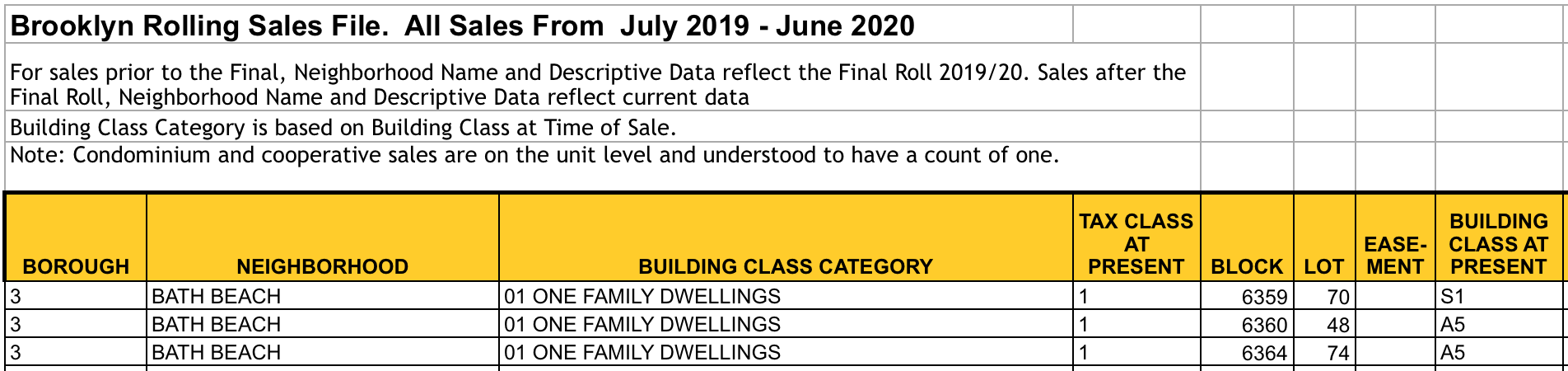The Brooklyn Excel file

Now let’s load the Brooklyn dataset into R from an Excel file. We’ll use the `readxl`package. We specify the function argument `skip = 4` because the row that we want to use as the header (i.e. column names) is actually row 5. We can ignore the first four rows entirely and load the data into R beginning at row 5. Here’s the code:

``````library(readxl) # Load Excel files
brooklyn <- read_excel("rollingsales_brooklyn.xls", skip = 4)
``````

Note we saved this dataset with the variable name `brooklyn` for future use.

## 4. View the Data with tidyr::glimpse()

The tidyverse offers a user-friendly way to view this data with the `glimpse()` function that is part of the `tibble` package. To use this package, we will need to load it for use in our current session. But rather than loading this package alone, we can load many of the tidyverse packages at one time. If you do not have the tidyverse collection of packages, install it on your machine using the following command in your R or R Studio session:

``````install.packages("tidyverse")
``````

Once the package is installed, load it to memory:

``````library(tidyverse)
``````

Now that `tidyverse` is loaded into memory, take a “glimpse” of the Brooklyn dataset:

``````glimpse(brooklyn)
## Observations: 20,185
## Variables: 21
## \$ BOROUGH <chr> "3", "3", "3", "3", "3", "3", "…
## \$ NEIGHBORHOOD <chr> "BATH BEACH", "BATH BEACH", "BA…
## \$ `BUILDING CLASS CATEGORY` <chr> "01 ONE FAMILY DWELLINGS", "01 …
## \$ `TAX CLASS AT PRESENT` <chr> "1", "1", "1", "1", "1", "1", "…
## \$ BLOCK <dbl> 6359, 6360, 6364, 6367, 6371, 6…
## \$ LOT <dbl> 70, 48, 74, 24, 19, 32, 65, 20,…
## \$ `EASE-MENT` <lgl> NA, NA, NA, NA, NA, NA, NA, NA,…
## \$ `BUILDING CLASS AT PRESENT` <chr> "S1", "A5", "A5", "A9", "A9", "…
## \$ ADDRESS <chr> "8684 15TH AVENUE", "14 BAY 10T…
## \$ `APARTMENT NUMBER` <chr> NA, NA, NA, NA, NA, NA, NA, NA,…
## \$ `ZIP CODE` <dbl> 11228, 11228, 11214, 11214, 112…
## \$ `RESIDENTIAL UNITS` <dbl> 1, 1, 1, 1, 1, 1, 1, 1, 2, 1, 1…
## \$ `COMMERCIAL UNITS` <dbl> 1, 0, 0, 0, 0, 0, 0, 0, 0, 0, 0…
## \$ `TOTAL UNITS` <dbl> 2, 1, 1, 1, 1, 1, 1, 1, 2, 1, 1…
## \$ `LAND SQUARE FEET` <dbl> 1933, 2513, 2492, 1571, 2320, 3…
## \$ `GROSS SQUARE FEET` <dbl> 4080, 1428, 972, 1456, 1566, 22…
## \$ `YEAR BUILT` <dbl> 1930, 1930, 1950, 1935, 1930, 1…
## \$ `TAX CLASS AT TIME OF SALE` <chr> "1", "1", "1", "1", "1", "1", "…
## \$ `BUILDING CLASS AT TIME OF SALE` <chr> "S1", "A5", "A5", "A9", "A9", "…
## \$ `SALE PRICE` <dbl> 1300000, 849000, 0, 830000, 0, …
## \$ `SALE DATE` <dttm> 2020-04-28, 2020-03-18, 2019-0…
``````

The `glimpse()` function provides a user-friendly way to view the column names and data types for all columns, or variables, in the data frame. With this function, we are also able to view the first few observations in the data frame. This data frame has 20,185 observations, or property sales records. And there are 21 variables, or columns.

#data science tutorials #beginner #r #r tutorial #r tutorials #rstats #tidyverse #tutorial #tutorials1599097440

## Data Visualization in R with ggplot2: A Beginner Tutorial

A famous general is thought to have said, “A good sketch is better than a long speech.” That advice may have come from the battlefield, but it’s applicable in lots of other areas — including data science. “Sketching” out our data by visualizing it using ggplot2 in R is more impactful than simply describing the trends we find.

This is why we visualize data. We visualize data because it’s easier to learn from something that we can see rather than read. And thankfully for data analysts and data scientists who use R, there’s a tidyverse package called ggplot2 that makes data visualization a snap!

In this blog post, we’ll learn how to take some data and produce a visualization using R. To work through it, it’s best if you already have an understanding of R programming syntax, but you don’t need to be an expert or have any prior experience working with ggplot2

#data science tutorials #beginner #ggplot2 #r #r tutorial #r tutorials #rstats #tutorial #tutorials